Multiplication of matrices

Chapter 3 Class 12 Matrices
Concept wise

Let’s look at some properties of multiplication of matrices.

1. Commutativity is not true:

AB ≠ BA

2. Zero matrix on multiplication

If AB = O,

then A ≠ O, B ≠ O is possible

3. Associative law:

(AB) C = A (BC)

4. Distributive law:

A (B + C) = AB + AC

(A + B) C = AC + BC

•

5. Multiplicative identity:

For a square matrix A

AI = IA = A

where I is the identity matrix of the same order as A.

Let’s look at them in detail

We used these matrices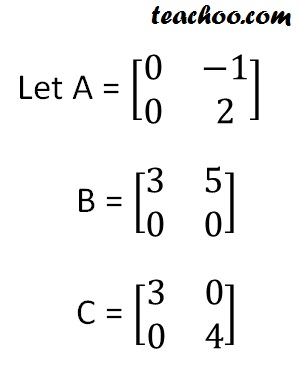## Commutativity in multiplication is not true

AB ≠ BA

Let’s solve them

AB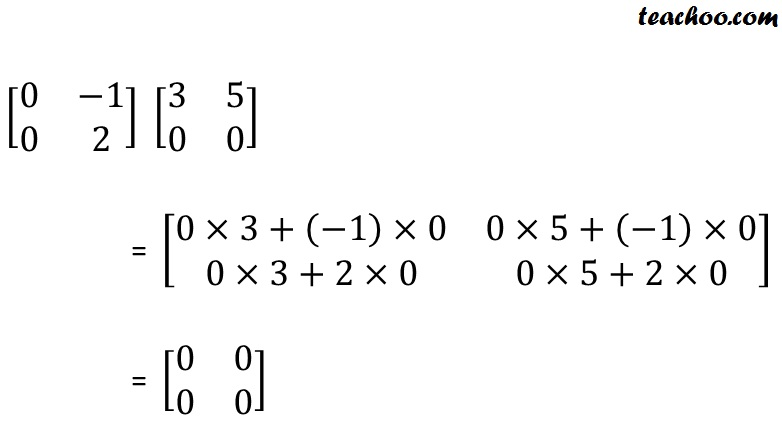BA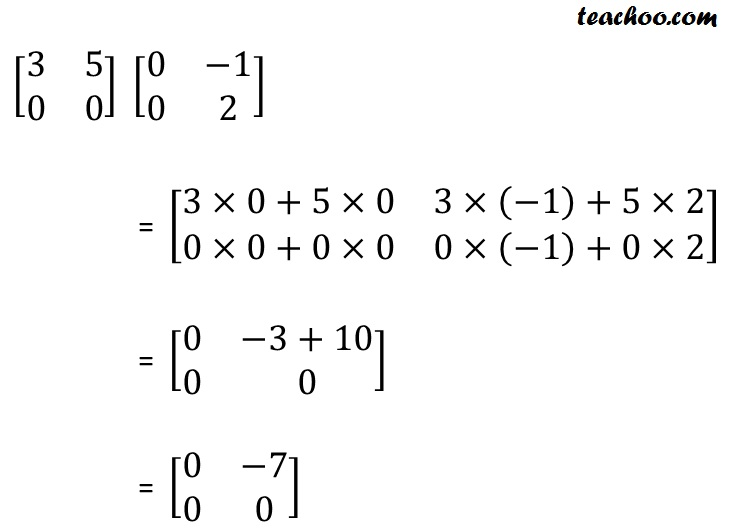Since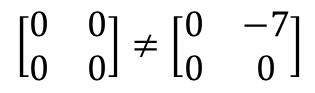∴ AB ≠ BA

## Zero matrix multiplication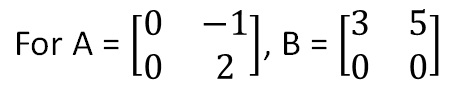We saw that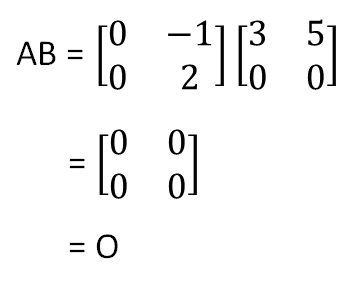So, AB = O

But A ≠ O & B ≠ O

Therefore,

If two matrices multiply to become zero matrix,

then it is not true that A = O or B = O

Note: This is different from numbers

If ab = 0,
then either a = 0 or b = 0

But this is not true for matrices

## Associative law

(AB) C = A (BC)

Let’s solve this

(AB) C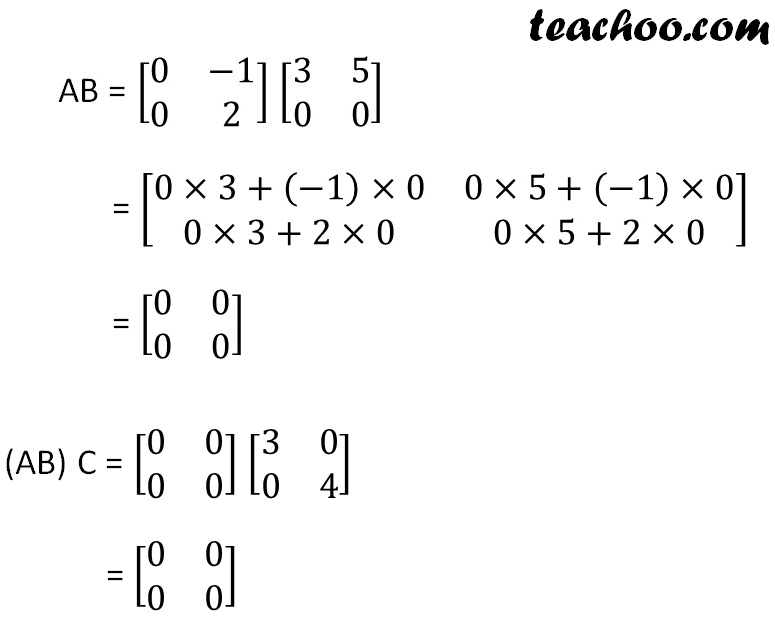Note: Any matrix multiplied to zero matrix is a zero matrix

(AB) C = O × C

= O

A (BC)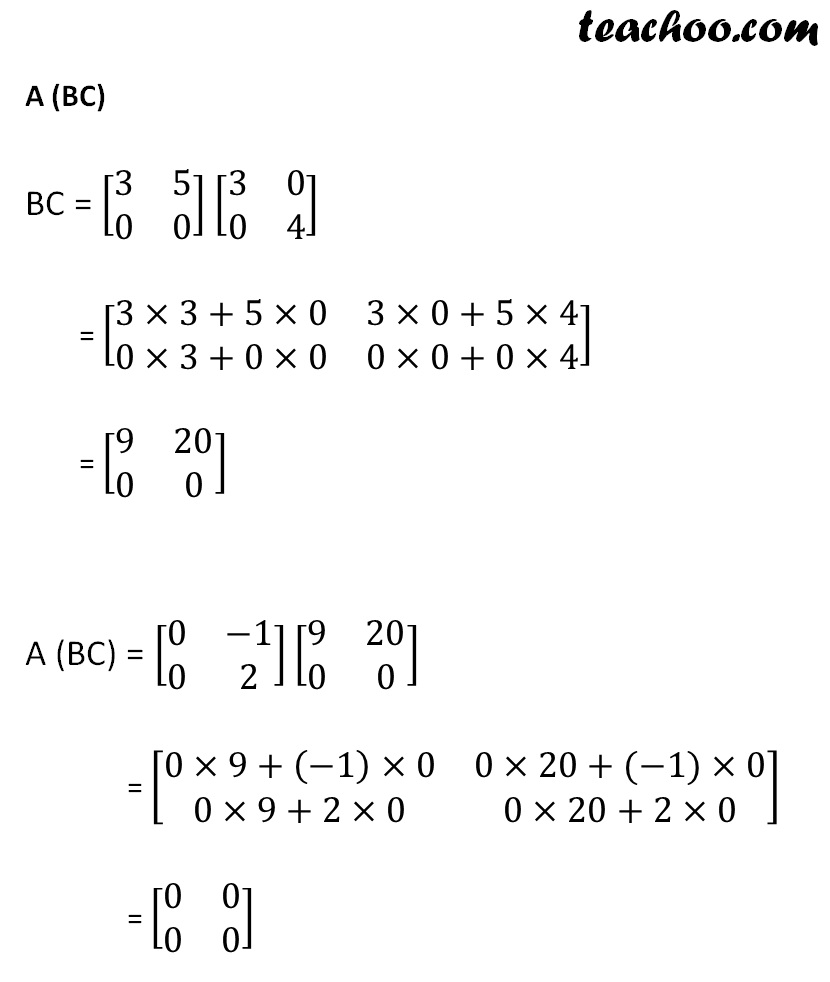.

Therefore,

(AB) C = A (BC)

## Distributive law

Distributive law says that -

• A (B + C) = AB + AC
• (A + B) C = AC + BC

Let’s prove both of them

### A (B + C) = AB + AC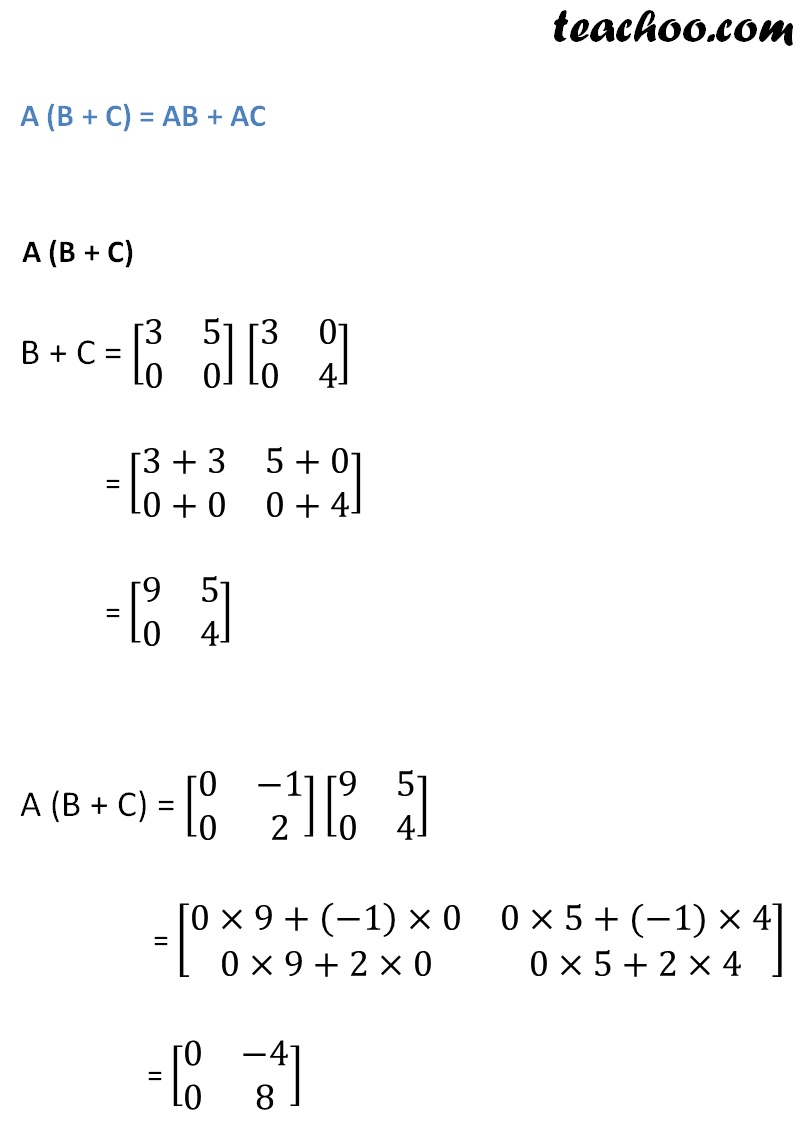### AB + AC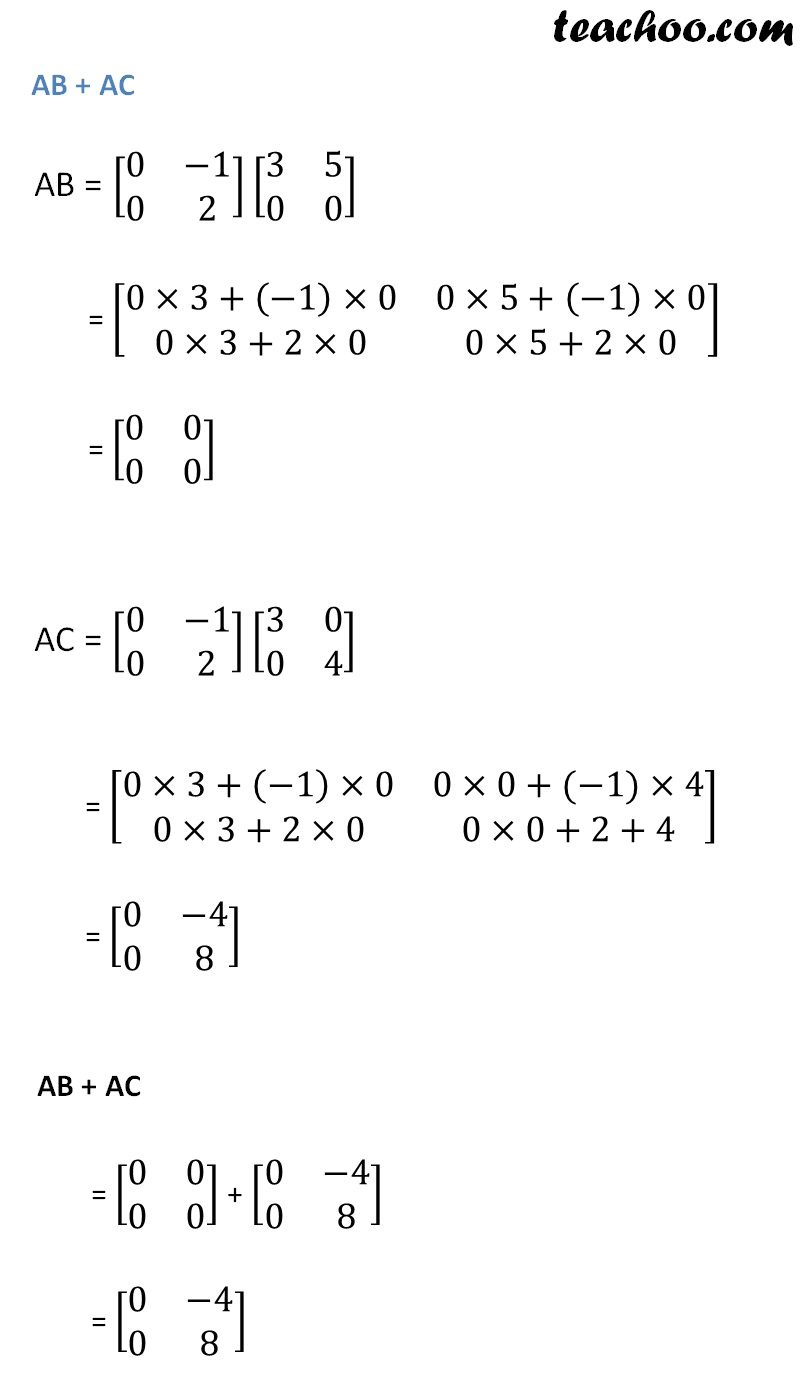Therefore,

A (B + C) = AB + AC

Let’s prove the next one

### (A + B) C = AC + BC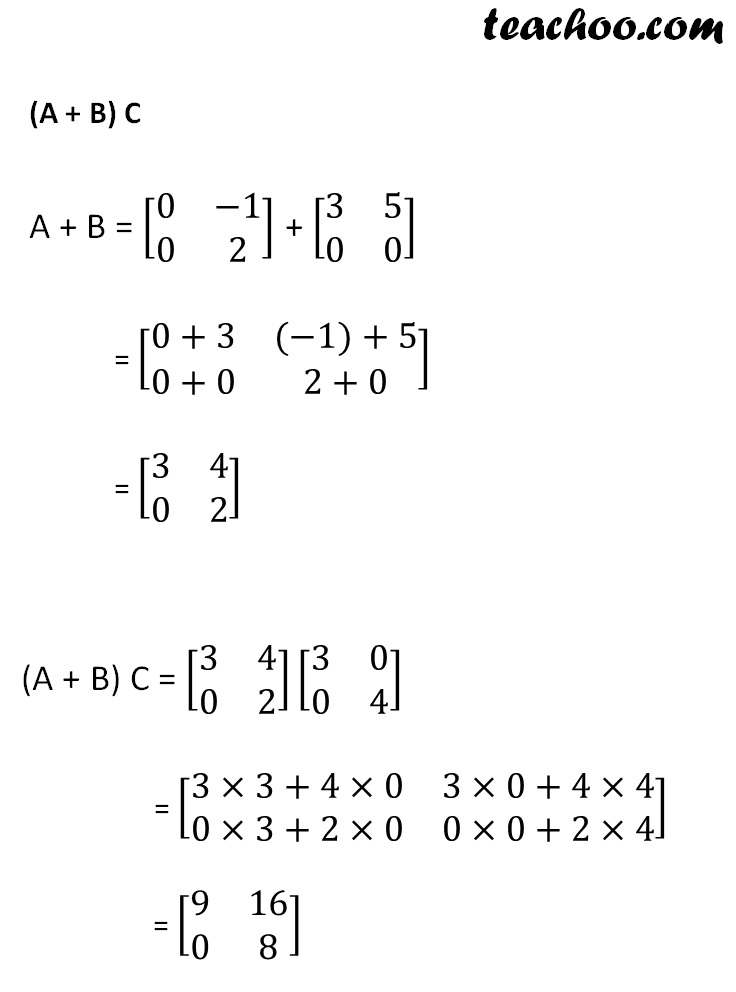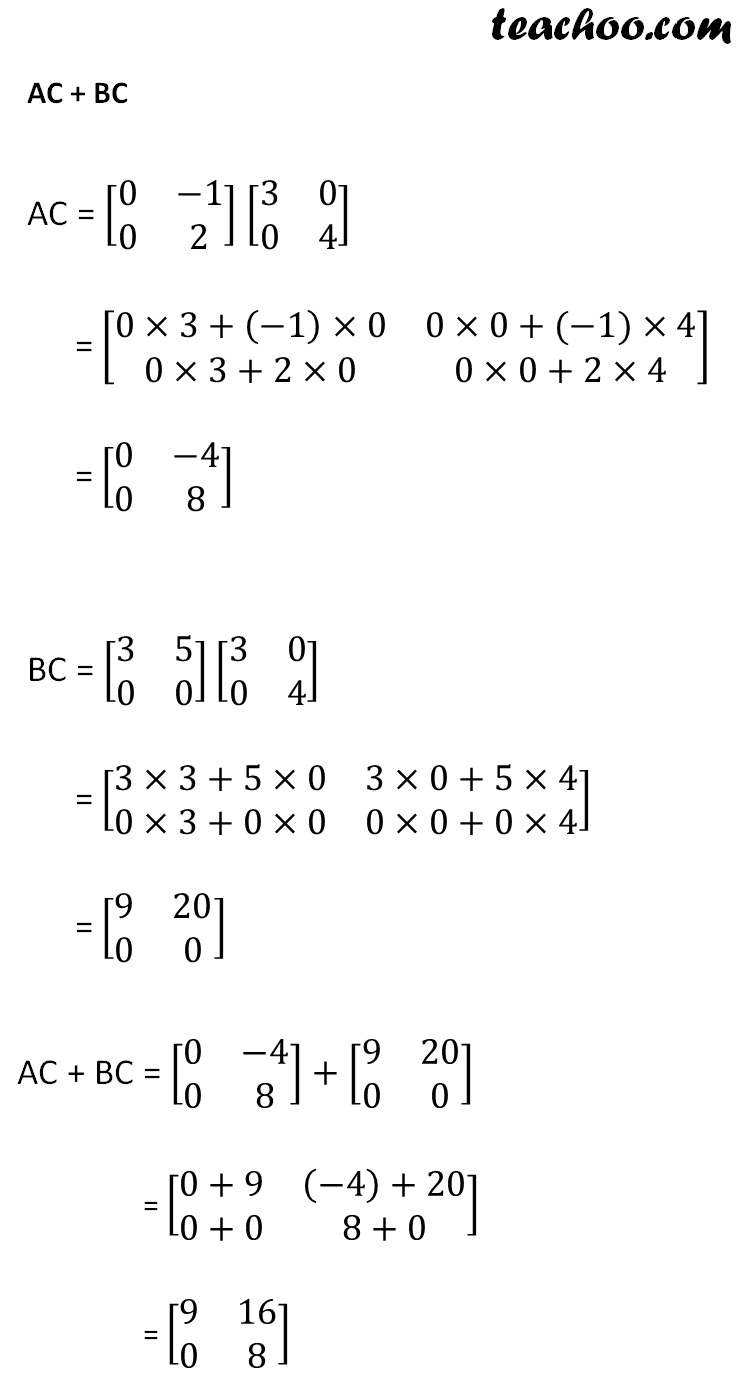Therefore,

(A + B) C = AC + BC

## Multiplicative Identity

For any square matrix A,

AI = IA = A

Where I is identity matrix of same order as A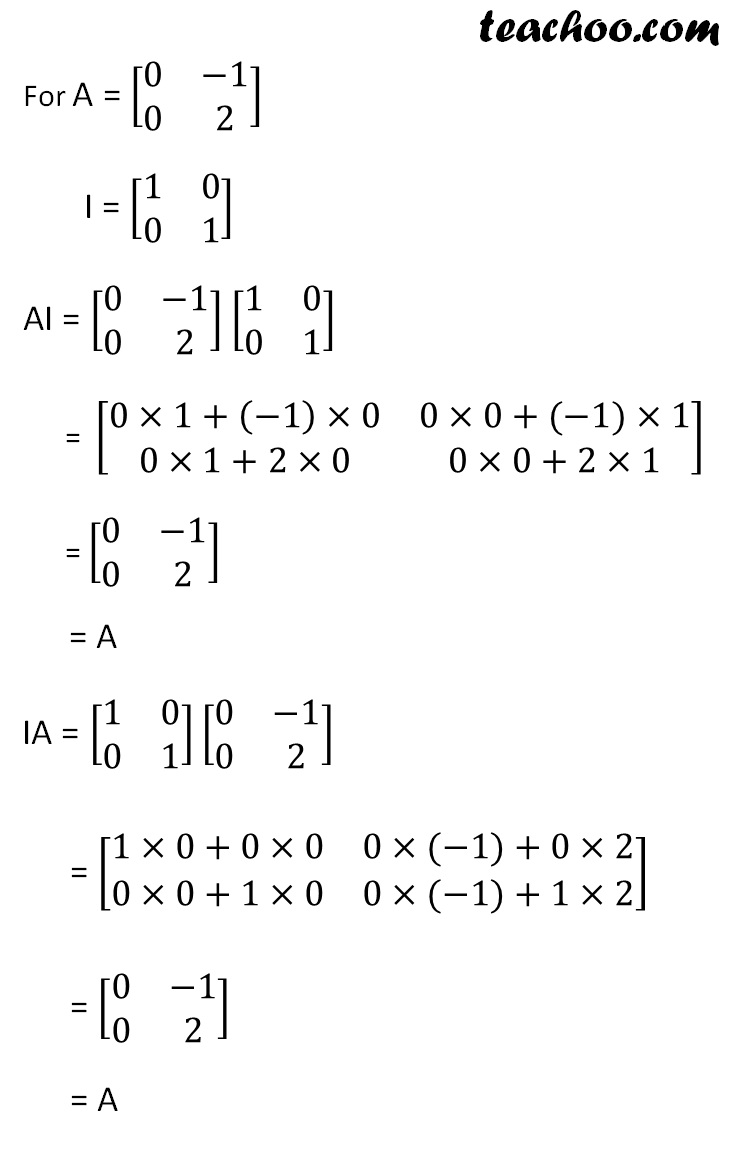Therefore,

AI = IA = A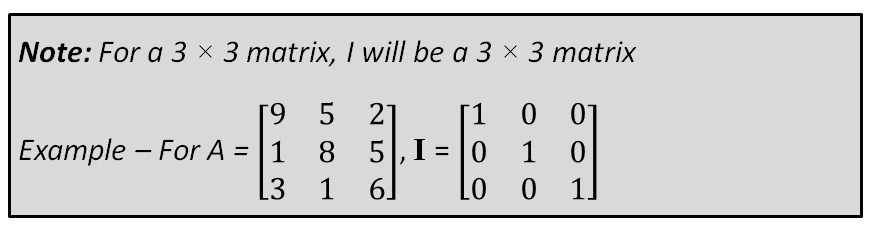Learn in your speed, with individual attention - Teachoo Maths 1-on-1 Class

### Transcript

Let A = [■8(0&−1@0&2)] B = [■8(3&5@0&0)] C = [■8(3&0@0&4)] AB [■8(0&−1@0&2)] [■8(3&5@0&0)] = [■8(0×3+(−1)×0&0×5+(−1)×0@0×3+2×0&0×5+2×0)] = [■8(0&0@0&0)] BA [■8(3&5@0&0)] [■8(0&−1@0&2)] = [■8(3×0+5×0&3×(−1)+5×2@0×0+0×0&0×(−1)+0×2)] = [■8(0&−3+10@0&0)] = [■8(0&−7@0&0)] Since [■8(0&0@0&0)]≠[■8(0&−7@0&0)] ∴ AB ≠ BA Zero matrix multiplication For A = [■8(0&−1@0&2)], B = [■8(3&5@0&0)] We saw that AB = [■8(0&−1@0&2)][■8(3&5@0&0)] = [■8(0&0@0&0)] = O (AB) C AB = [■8(0&−1@0&2)] [■8(3&5@0&0)] = [■8(0×3+(−1)×0&0×5+(−1)×0@0×3+2×0&0×5+2×0)] = [■8(0&0@0&0)] (AB) C = [■8(0&0@0&0)] [■8(3&0@0&4)] = [■8(0&0@0&0)] A (BC) BC = [■8(3&5@0&0)] [■8(3&0@0&4)] = [■8(3×3+5×0&3×0+5×4@0×3+0×0&0×0+0×4)] = [■8(9&20@0&0)] A (BC) = [■8(0&−1@0&2)][■8(9&20@0&0)] = [■8(0×9+(−1)×0&0×20+(−1)×0@0×9+2×0&0×20+2×0)] = [■8(0&0@0&0)] Therefore, (AB) C = A (BC) A (B + C) B + C = [■8(3&5@0&0)] [■8(3&0@0&4)] = [■8(3+3&5+0@0+0&0+4)] = [■8(9&5@0&4)] A (B + C) = [■8(0&−1@0&2)][■8(9&5@0&4)] = [■8(0×9+(−1)×0&0×5+(−1)×4@0×9+2×0&0×5+2×4)] = [■8(0&−4@0&8)] AB + AC AB = [■8(0&−1@0&2)][■8(3&5@0&0)] = [■8(0×3+(−1)×0&0×5+(−1)×0@0×3+2×0&0×5+2×0)] = [■8(0&0@0&0)] AC = [■8(0&−1@0&2)][■8(3&0@0&4)] = [■8(0×3+(−1)×0&0×0+(−1)×4@0×3+2×0&0×0+2+4)] = [■8(0&−4@0&8)] AB + AC = [■8(0&0@0&0)] + [■8(0&−4@0&8)] = [■8(0&−4@0&8)] Therefore, A (B + C) = AB + AC Let’s prove the next one (A + B) C A + B = [■8(0&−1@0&2)] + [■8(3&5@0&0)] = [■8(0+3&(−1)+5@0+0&2+0)] = [■8(3&4@0&2)] (A + B) C = [■8(3&4@0&2)][■8(3&0@0&4)] = [■8(3×3+4×0&3×0+4×4@0×3+2×0&0×0+2×4)] = [■8(9&16@0&8)] AC + BC AC = [■8(0&−1@0&2)] [■8(3&0@0&4)] = [■8(0×3+(−1)×0&0×0+(−1)×4@0×3+2×0&0×0+2×4)] = [■8(0&−4@0&8)] BC = [■8(3&5@0&0)][■8(3&0@0&4)] = [■8(3×3+5×0&3×0+5×4@0×3+0×0&0×0+0×4)] = [■8(9&20@0&0)] AC + BC = [■8(0&−4@0&8)]+[■8(9&20@0&0)] = [■8(0+9&(−4)+20@0+0&8+0)] = [■8(9&16@0&8)] Therefore, (A + B) C = AC + BC For A = [■8(0&−1@0&2)] I = [■8(1&0@0&1)] AI = [■8(0&−1@0&2)] [■8(1&0@0&1)] = [■8(0×1+(−1)×0&0×0+(−1)×1@0×1+2×0&0×0+2×1)] = [■8(0&−1@0&2)] = A IA = [■8(1&0@0&1)][■8(0&−1@0&2)] = [■8(1×0+0×0&0×(−1)+0×2@0×0+1×0&0×(−1)+1×2)] = [■8(0&−1@0&2)] = A Note: For a 3 × 3 matrix, I will be a 3 × 3 matrix Example – For A = [■8(9&5&2@1&8&5@3&1&6)], I = [■8(1&0&0@0&1&0@0&0&1)]